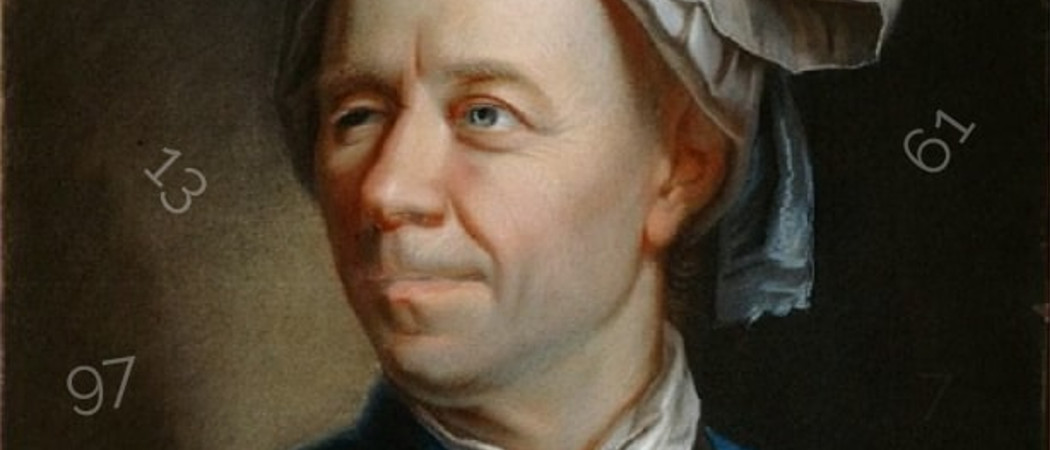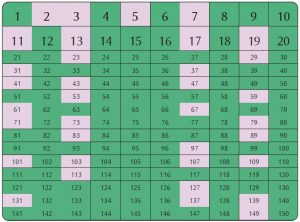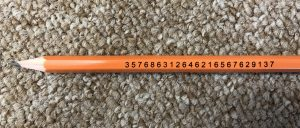# Primes à la Euler

Infinitely many primes ending in 1, 3, 7 and 9 proved in typically Eulerian style.Anyone with a more than a passing interest in numbers knows that there are infinitely many primes.If we highlight them in a grid like this, it is clear that the last digit of any prime must be either 1, 3, 7 or 9 (except for 2 and 5 of course). But there seems to be no good reason for primes to prefer any one of these four possibilities over any other. It is natural therefore to conjecture that

There are infinitely many primes whose last digit is $b$ for $b$ equal to any of 1, 3, 7, or 9.

Unless you have seen this before you probably don’t know how to prove it. When stuck trying to prove something and you don’t know what to do, it is often a good tactic to first try to prove something simpler than, but related to, what you really want to prove.

One way to simplify this particular problem is to notice the following: besides the exceptions 2 and 5, when we divide a prime $p$ by 10 there are four possibilities for the remainder, 1, 3, 7 or 9, which we write as $p \equiv 1, 3, 7 \text{ or } 9 \text{ (mod } 10 ).$

When we divide an odd prime by 4 though, there are only two possibilities, $1$ or $3$. So we might start by trying to prove that there are infinitely many primes $p$ such that $p \equiv b \text{ (mod 4)}$ for any $b$ equal to 1 or 3. This just means that the remainder when we divide $p$ by 4 is $b$. Let’s now see how Euler might have thought about this problem by looking at how he proved that there are infinitely many primes.

## Primes, infinite series and π

$$\sum_{n=1}^{\infty}\frac{1}{n} = 1 + \frac{1}{2} + \frac{1}{3} + \frac{1}{4} + \frac{1}{5} + \cdots = \prod_{\text{ primes } p }\frac{1}{1-\frac{1}{p}}.$$
Knowing that the left hand side diverges to $\infty$, we see immediately that the right hand side cannot be a finite product. And that’s it! The right side cannot be a finite product–so there are infinitely many primes! That’s Euler’s proof. But how do you prove the identity? Simple. Expand each factor of the right hand side into a geometric sum to get
$$\prod_{p}\left(1 + \frac{1}{p} + \frac{1}{p\hspace{1pt}^2} + \frac{1}{p\hspace{1pt}^3} \cdots \right).$$
Multiplying out the product involves choosing a $1/p\hspace{1pt}^k$ from each factor, multiplying these $1/p\hspace{1pt}^k$ together and summing over all the ways of choosing the value of $k$. The only resulting terms which are non-zero are those in which we pick $k$ to be non-zero for only finitely many primes and $k=0$ for all other primes. Doing so, we get $1/n$ for each positive integer $n$ precisely once, just as we wanted. This is a consequence of the fundamental theorem of arithmetic which says that each integer can be written as a product of primes in a unique way (up to the order of the primes). It is well worth thinking this through so that it makes sense because it is the most crucial part of the argument.

Euler used his product formula to prove even more. By taking the logarithm of $\infty$ to get $\infty$ and converting the infinite product into an infinite sum (this is not rigorous mathematics by today’s standards but Euler wasn’t one to let pedantic details spoil a beautiful argument!)
$$\infty = \log \left(\prod_{\text{ primes } p }\frac{1}{1-\frac{1}{p}} \right) = – \sum_{p}\log\left(1-\frac{1}{p} \right).$$
After expanding log using the power series
$$-\log(1-x) = x + \frac{x^2}{2} + \frac{x^3}{3} + \frac{x^4}{4} + \cdots,$$
valid for all $-1<x<1$, Euler gets
$$\infty = \sum_{p}\frac{1}{p} + \frac{1}{2}\sum_{p}\frac{1}{p\hspace{1pt}^2} + \frac{1}{3}\sum_{p}\frac{1}{p\hspace{1pt}^3} + \cdots.$$
Most of these sums are bounded. In fact,
$$\frac{1}{2}\sum_{p}\frac{1}{p\hspace{1pt}^2} + \frac{1}{3}\sum_{p}\frac{1}{p\hspace{1pt}^3} + \cdots < \sum_{p} \sum_{n = 2}^{\infty}\frac{1}{p\hspace{1pt}^n} = \sum_{p}\frac{1}{p\hspace{1pt}(p-1)} < \sum_{n=1}^{\infty}\frac{1}{n\hspace{1pt}^2} < \infty.$$
So we have the astonishing conclusion that
$$\sum_{p}\frac{1}{p} = \infty.$$
Remember that we would like to show that the primes are in some sense split evenly between the two classes 1 (mod 4) and 3 (mod 4) – and in particular that there are infinitely many of each type. Inspired by Euler’s argument involving infinite series, we next introduce the following alternating series
$$A = -\frac{1}{3} + \frac{1}{5} – \frac{1}{7} – \frac{1}{11} + \frac{1}{13} – \frac{1}{17} + \ldots =\sum_{p \neq 2 }\frac{(-1)^{(p-1)/2}}{p}$$
which has a plus sign in front of those primes which are 1 (mod 4) and a minus sign in front of those primes which are 3 (mod 4). The prime 2 doesn’t really feel like it belongs in this series and in particular it is not clear what the sign for $1/2$ ought to be. To avoid having to make an arbitrary choice we will just not include the prime 2.A pencil showing the largest `left-truncatable’ prime, created by Maths Inspiration. Image: Luciano Rila

Our strategy then is to prove that the series $A$ converges. If we could, it would then follow immediately from $\sum{1/p} = \infty$ that there are infinitely many primes of each type, 1 and 3 mod 4. Because if there were only finitely many of one of these types, the series $A$ would diverge.

Taking a cue from Euler’s original argument, we can add in the prime powers without changing whether or not the series converges (because, as we have seen, the sum of the reciprocals of the prime powers converges). So expanding the logarithm into an infinite series, it follows just as before that $A$ converges if and only if
$$-\sum_{p \neq 2}\log\left(1-\frac{(-1)^{(p-1)/2}}{p}\right)$$
converges. Exponentiating, this converges if and only if
$$\prod_{p \neq 2}\frac{1}{1-\frac{(-1)^{(p-1)/2}}{p}}$$
converges to something non-zero. We can expand this infinite product much like before by first writing each factor as a geometric sum
$$\prod_{p \neq 2}\left(1+ \frac{(-1)^{(p-1)/2}}{p} + \frac{1}{p\hspace{1pt}^2} + \frac{(-1)^{(p-1)/2}}{p\hspace{1pt}^3} + \cdots \right).$$
Expanding the product is a bit harder this time but not too bad. It is
$$\prod_{p \neq 2}\left(1+ \frac{(-1)^{(p-1)/2}}{p} + \frac{1}{p\hspace{1pt}^2} + \frac{(-1)^{(p-1)/2}}{p\hspace{1pt}^3} + \cdots \right) = 1 – \frac{1}{3} + \frac{1}{5} – \frac{1}{7} + \frac{1}{9} – \frac{1}{11} + \cdots$$
now with only odd terms and every other term having a minus sign. This one is more difficult because we need to keep track of all the minus signs. Multiplying out the product, we get $\pm1/n$ for each odd integer $n$ exactly once for some choice of sign. There are no even integers as we have excluded the prime 2. An integer appears with a minus sign if and only if it has an odd number of prime factors which are $3 \text{ (mod 4)}$. This is exactly the right hand side though since such integers are exactly those which are themselves $3 \text{ (mod 4)}$. You might want to stop to check this last point makes sense.

But wait. We recognise this (don’t we) as Leibniz’s famous series
$$\frac{\mathrm{\pi}}{4} = 1 – \frac{1}{3} + \frac{1}{5} – \frac{1}{7} + \frac{1}{9} – \frac{1}{11} + \cdots$$
which is certainly finite.

So, there we have it
$$\sum_{p \neq 2}\frac{(-1)^{(p-1)/2}}{p} = \text{“something finite”}$$
and we proved above that
$$\sum_{p\equiv 1 \text{ (mod 4)}}\frac{1}{p} + \sum_{p\equiv 3 \text{ (mod 4)}}\frac{1}{p} = \infty$$
because leaving out the prime 2 doesn’t change whether or not the series converges.
By adding and subtracting these two equations we conclude that.
$$\sum_{p\equiv 1 \text{ (mod 4)}}\frac{1}{p} = \infty \:\:\:\:\:\: \text{ and } \:\:\:\:\:\: \sum_{p\equiv 3 \text{ (mod 4)}}\frac{1}{p} = \infty.$$
What an astounding thing to follow from an infinite series for $\mathrm{\pi}$!

## The proof

As pleased as we are with this dramatic conclusion, it doesn’t solve our original problem. We wanted to show that for each $b=$ 1, 3, 7, 9, there are infinitely many primes whose last digit is $b$. We can rewrite this condition by noticing the following equivalences which hold for all odd numbers $p$,
\begin{align*}
p \equiv 1 \text{ (mod 10)} &\Leftrightarrow p \equiv 1 \text{ (mod 5)} \\
p \equiv 3 \text{ (mod 10)} &\Leftrightarrow p \equiv 3 \text{ (mod 5)} \\
p \equiv 7 \text{ (mod 10)} &\Leftrightarrow p \equiv 2 \text{ (mod 5)} \\
p \equiv 9 \text{ (mod 10)} &\Leftrightarrow p \equiv 4 \text{ (mod 5)}.
\end{align*}

Convince yourself this is true by trying a few examples. So can we do for 5 what we just did for 4? It will be a bit harder this time because there are 4 remainder options for our primes rather than just two. Let’s first take some time to reflect on what made the argument work before we try to generalise it.

1. We had an infinite convergent series that factored into primes and could somehow distinguish between primes in different remainder classes modulo 4.
2. We took logarithms and “solved for” the two sums $\sum_{p \equiv 1 \text{ (mod 4)}}1/p$ and $\sum_{p \equiv 3 \text{ (mod 4)}}1/p$ by taking a linear combination with the logarithm of Euler’s product formula.
3. To deduce that these sums over primes were infinite, it was important that the original series not only converged, but that it converged to something non-zero. We needed it to be non-zero so we could take the logarithm and get a finite answer. It wasn’t important what the answer was, just that it was finite.

Point 1 suggests (or rather, it suggested to Dirichlet) that we assign coefficients $\chi(n)$ to each integer $n$ which only depend on the remainder of $n$ when divided by 5. These coefficients are to be used to detect which class the primes belongs to. They should also give rise to a nice convergent series which can be factored into a product over all primes
$$\sum_{n = 1}^{\infty}\frac{\chi(n)}{n} = \prod_{p}\text{factor(p)}.$$
We will be able to factor the series just as before if the coefficients have the special property
$$\chi(mn) = \chi(m)\chi(n) \text{ for all positive integers } m \text{ and } n.$$
If $\chi$ has this special property then we can write
$$\sum_{n=1}^{\infty}\frac{\chi(n)}{n} = \prod_{p}\frac{1}{1-\frac{\chi(p)}{p}}.$$
The proof is almost exactly the same as before. This multiplicativity property is quite restrictive, especially if we also require $\chi(n)$ to only depend on the remainder of $n$ by 5. First of all, if we don’t want our $\chi(n)$ to equal zero for all $n$ then we must have $\chi(1) = 1$ since $\chi(n) =\chi(n\cdot1) = \chi(n)\cdot \chi(1)$ so $\chi(1) \neq 1$ implies $\chi(n) = 0$ for all $n$. If we set $\chi(2) = \alpha$, say, $\chi(4)$ is determined to be $\chi(4)=\chi(2\cdot2) = \chi(2) \cdot \chi(2) = \alpha^2$. Similarly, the value of $\chi(3)$ is determined by $\chi(3) = \chi(4\cdot2) = \chi(4) \cdot \chi(2) = \alpha^3$. Here we used that $4\cdot 2 = 8 \equiv 3\text{(mod 5)}$. So we just need to decide on a value for $\alpha$ and $\chi(0)$. Recalling what happened before, we will set $\chi(0)$ to be zero. 5 is the only prime in this remainder class anyway so if the point of these coefficients is to detect primes, they ought to be zero on this class. We don’t actually have much choice for $\alpha$ since $1 = \chi(1)= \chi(3\cdot 2) = \chi(3) \cdot \chi(2) = \alpha^4$. Therefore, $\alpha$ must equal $1$, $i$, $-1$ or $-i$. Each of these choices of $\alpha$ gives a different $\chi$ which we will denote $\chi_\alpha$. In table format:Each $\chi$ gives us a different series
\begin{align*}
\sum_{n=1}^{\infty}\frac{\chi_1(n)}{n} &= \frac{1}{1} + \frac{1}{2} + \frac{1}{3} + \frac{1}{4} + \frac{1}{6} + \frac{1}{7} + \frac{1}{8} + \frac{1}{9} + \cdots\\
\sum_{n=1}^{\infty}\frac{\chi_{i}(n)}{n} &= \frac{1}{1} + \frac{i}{2} -\frac{1}{3} – \frac{i}{4} + \frac{1}{6} + \frac{i}{7} – \frac{1}{8} – \frac{i}{9} + \cdots\\
\sum_{n=1}^{\infty}\frac{\chi_{-1}(n)}{n} &= \frac{1}{1} – \frac{1}{2} – \frac{1}{3} + \frac{1}{4} + \frac{1}{6} – \frac{1}{7} – \frac{1}{8} + \frac{1}{9} + \cdots\\
\sum_{n=1}^{\infty}\frac{\chi_{-i}(n)}{n} &= \frac{1}{1} – \frac{i}{2} + \frac{i}{3} – \frac{1}{4} + \frac{1}{6} – \frac{i}{7} + \frac{i}{8} – \frac{1}{9} + \cdots
\end{align*}

This is nice because point 2 above suggests that we need \emph{four} series to solve for our \emph{four} unknowns $\sum_{p \equiv b \text{ (mod 5)}}1/p$ with $b = 1,2,3$ or 4. Let us look at each of these four series in turn and see if they have the properties we want.

Case 1: $\alpha = 1$. This one diverges and is the one that tells us that $\sum_{p}1/p = \infty$. In some sense this is the most important one because it is the one that tells us we have infinitely many primes when we include all remainder classes.

Case 2: $\alpha = i$. This one involves the complex number $i$ and it is natural to look at its real and imaginary parts separately. Remember that we want to show that it converges to a finite non-zero number but we are not overly interested in what that number is, so long as it isn’t 0 or $\infty$. This will happen provided the real and imaginary parts both converge to some finite limits that are not both zero. This in turn is fairly easy to prove by grouping the positive and negative terms together. We will do the real part and invite the reader to fill in the details for the imaginary part.
\begin{align*}
\frac{1}{1} -\frac{1}{3} + \frac{1}{6} – \frac{1}{8} + \frac{1}{11} – \frac{1}{13}+\cdots &= \sum_{k = 1}^{\infty}\left(\frac{1}{5k – 4} – \frac{1}{5k-2}\right) \\
&= \sum_{k=1}^{\infty}\frac{2}{(5k-4)(5k-2)} < \sum_{n=1}^{\infty}\frac{2}{n\hspace{1pt}^2} < \infty.
\end{align*}
Notice that each term $\frac{2}{(5k – 4)(5k-2)}$ is positive so the sum is certainly not zero.

Case 3: $\alpha = -1$. We can do this by collecting the terms into groups of 4 and using a little algebra.
\begin{align*}
\frac{1}{1} &-\frac{1}{2} – \frac{1}{3} + \frac{1}{4} + \frac{1}{6} – \frac{1}{7} – \frac{1}{8} + \frac{1}{9} + \cdots = \sum_{k=1}^{\infty}\left( \frac{1}{5k-4}-\frac{1}{5k-3}-\frac{1}{5k-2}+\frac{1}{5k-1} \right) \\
&= \sum_{k=1}^{\infty}\tfrac{(5k-3)(5k-2)(5k-1)-(5k-4)(5k-2)(5k-1)-(5k-4)(5k-3)(5k-1)+(5k-3)(5k-2)(5k-1)}{(5k-4)(5k-3)(5k-2)(5k-1)} \\
&= \sum_{k=1}^{\infty}\frac{20k – 2}{(5k-4)(5k-3)(5k-2)(5k-1)} < \infty.
\end{align*}
Again, each term $\frac{20k – 2}{(5k-4)(5k-3)(5k-2)(5k-1)}$ is positive so whatever this sum is, it is positive.

Case 4: $\alpha =-i$. This one is very similar to case 2 so we will leave the details to the interested reader to fill in for herself.

Now that we know our series converge to non-zero limits we can take logarithms to convert our products over primes into sums over primes. Expanding log into an infinite series and bounding the terms involving prime powers exactly as before we get the following four equations:

$$\sum_{p}\frac{\chi_1(p)}{p} = \infty, \:\:\: \sum_{p}\frac{\chi_i(p)}{p} = \text{“finite”}, \:\:\: \sum_{p}\frac{\chi_{-1}(p)}{p} = \text{“finite”}, \:\:\: \sum_{p}\frac{\chi_{-i}(p)}{p} = \text{“finite”},$$
which we can write more explicitly as
\begin{align*}
\lim_{n \rightarrow \infty}\sum_{\substack{ p \leq n \\ p \equiv 1\text{ (mod 5)}}}\frac{1}{p} + \sum_{\substack{ p \leq n \\ p \equiv 2\text{ (mod 5)}}}\frac{1}{p} + \sum_{\substack{ p \leq n \\ p \equiv 3\text{ (mod 5)}}}\frac{1}{p} + \sum_{\substack{ p \leq n \\ p \equiv 4\text{ (mod 5)}}}\frac{1}{p} &= \infty \\
\lim_{n \rightarrow \infty}\sum_{\substack{ p \leq n \\ p \equiv 1\text{ (mod 5)}}}\frac{1}{p} + \sum_{\substack{ p \leq n \\ p \equiv 2\text{ (mod 5)}}}\frac{i}{p} – \sum_{\substack{ p \leq n \\ p \equiv 3\text{ (mod 5)}}}\frac{i}{p} – \sum_{\substack{ p \leq n \\ p \equiv 4\text{ (mod 5)}}}\frac{1}{p} &= \text{“finite”} \\
\lim_{n \rightarrow \infty}\sum_{\substack{ p \leq n \\ p \equiv 1\text{ (mod 5)}}}\frac{1}{p} – \sum_{\substack{ p \leq n \\ p \equiv 2\text{ (mod 5)}}}\frac{1}{p} – \sum_{\substack{ p \leq n \\ p \equiv 3\text{ (mod 5)}}}\frac{1}{p} + \sum_{\substack{ p \leq n \\ p \equiv 4\text{ (mod 5)}}}\frac{1}{p} &= \text{“finite”} \\
\lim_{n \rightarrow \infty}\sum_{\substack{ p \leq n \\ p \equiv 1\text{ (mod 5)}}}\frac{1}{p} – \sum_{\substack{ p \leq n \\ p \equiv 2\text{ (mod 5)}}}\frac{i}{p} + \sum_{\substack{ p \leq n \\ p \equiv 3\text{ (mod 5)}}}\frac{i}{p} – \sum_{\substack{ p \leq n \\ p \equiv 4\text{ (mod 5)}}}\frac{1}{p} &= \text{“finite”}.
\end{align*}
That’s a bit messy so let’s introduce the notation $S_b(n) = \sum_{\substack{ p \leq n \\ p \equiv b \text{ (mod 5)}}}1/p$ for $b = 1, 2, 3, 4$ and rewrite it as
\begin{align*}
\lim_{n \rightarrow \infty} S_1(n) + S_2(n) + S_3(n) + S_4(n) &= \infty \\
\lim_{n \rightarrow \infty} S_1(n) + i S_2(n) -i S_3(n) – S_4(n) &= \text{“finite”} \\
\lim_{n \rightarrow \infty} S_1(n) – S_2(n) – S_3(n) + S_4(n) &= \text{“finite”} \\
\lim_{n \rightarrow \infty} S_1(n) -i S_2(n) +i S_3(n) – S_4(n) &= \text{“finite”}.
\end{align*}
This looks like a simple system of four linear equations in four unknowns, but it involves limits and infinity and one must not be tempted into taking the limits too soon and writing nonsense like $\infty +i \infty -i\infty -\infty = \text{“finite”}$. We leave the task of carefully deducing that $\sum_{p\equiv b \text{ (mod 5)}}1/p = \lim_{n \rightarrow \infty} S_b(n) = \infty$ for each $b = 1, 2, 3, 4$ as an exercise. If successful, the reader will have finished the proof of our original conjecture, and proved that there are infinitely many primes ending in each of 1, 3, 7, and 9!